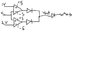# LED Circuit Design: Create w/1 Power Supply & 50mA Max

• Engineering
• wany
In summary, the conversation discusses designing a circuit that can take in a range of input voltages and light up an LED accordingly. The circuit must use one power supply and cannot draw more than 50mA. A potential solution is suggested using op-amps and resistors, and the final design is tested and confirmed to work.

## Homework Statement

I need to create a circuit that will take in a Vin, and then for a Vin of less than or equal to 1V, or a Vin greater than or equal to 2V, an led will light up.

For this I can only use one power supply of +-5V, and the circuit cannot draw more than 50mA.

## Homework Equations

Basically, its Vin goes to the Circuit which goes to the led

## The Attempt at a Solution

I'm having a hard time imagining this, but I was thinking that this will probably require an op-amp where the power supply is connected to it at +5V on one terminal and -5V on the other. Then I would need this opamp for the Vin, to output a negative value if Vin is between 1V and 2V; and positive otherwise.

However, I am not sure how to do that.

any help would be appreciated.

I seem to recall a problem here very similar to this from 4 or 5 days back. It probably used two comparators.

Ok, so I looked into that. So i decided to draw this out. Does my drawing appear to be correct.

#### Attachments

•Window Comparators.jpg
11.8 KB · Views: 828
Thinking about the 50mA limit, would I need to add a resistor after each opamp in front of each diode. Seeing that the PS is +-5V, so using V=IR, R=V/I=5/.05=100 ohms

So would using a 100 ohm resistor in front of each diode work. Then the two branches meet up and go to the led.

Last edited:
wany said:
Thinking about the 50mA limit, would I need to add a resistor after each opamp in front of each diode. Seeing that the PS is +-5V, so using V=IR, R=V/I=5/.05=100 ohms

So would using a 100 ohm resistor in front of each diode work. Then the two branches meet up and go to the led.
The word "work" can carry a number of connotations.If you are designing for a target current through the LED, then you need to take into account the voltage drops across the diodes and LED. Does your LED even need 50mA?

"The circuit cannot draw more than 50mA" might mean that the op-amps plus the indicator LED in total must not exceed 50mA draw. So best to design for a small but adequate LED current, and check the data sheets for your dual comparator chip to see what it will draw. Some op-amps or comparators will draw less current than others.

What you have looks promising. You have one power supply, so where are you going to obtain the 2v and 1v? Your diodes form an OR gate and isolate the output of one comparator from the other.

Alright, so would I need to have feedback for each comparator in order to get the 1v and the 2v?

wany said:
Alright, so would I need to have feedback for each comparator in order to get the 1v and the 2v?
Just use potential dividers across the 5v to obtain 1v and 2v. Often circuits do use positive feedback, but you are not asked to here.

Oh ok. So would I split the output from the 5V power supply into three branches:
one going directly to the opamp, another going through a resistor of X ohms then providing 2v, and a third going through a resistor of Y ohms then providing 1v.

Each potential divider involves a pair of resistors, not a single resistor.

To demonstrate a working circuit, you'll probably use a variable resistor across the 0..+5 rails to obtain an input that can be swung over 0 to 5v to test you have the correct triggering levels of your comparators. Is this what you meant as going directly to the op-amp?

I'm still not sure that you understand the op-amps require power to run them, quite separate to their signal inputs, even though this is not shown on normal circuit diagrams.

You won't get 2v and 1v that way. For a voltage divider you need two resistors. One goes to +5v, the other to ground, and you tap off in the middle.

Thanks a lot for your help, you I realized that after I drew that picture, but we set it up in the lab and i got it working. Thanks a lot once again.

Wait, would you connect the LED to the vOUT of both of the op amps?

hayniesk said:
Wait, would you connect the LED to the vOUT of both of the op amps?
Hi hayniesk. http://img96.imageshack.us/img96/5725/red5e5etimes5e5e45e5e25.gif [Broken]

This thread you just posted carried its final message two years ago. Was there something new you wanted to ask about it?

Last edited by a moderator: